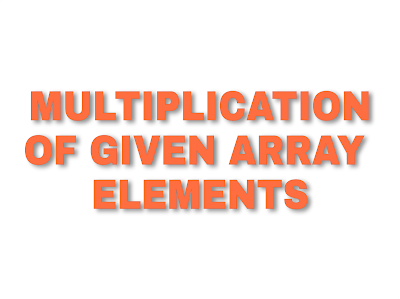Lompat ke konten Lompat ke sidebar Lompat ke footer

# Java Program to Print Multiplication of Given Array ElementsIn previous post we learnt how to print Sum of given array elements. In this post we will learn how to print multiplication of given array elements.

### Step by step logic of the given program:

1. Accept array limit from user store it in variable say lim.

2. Accept elements from user. Run a for loop from to arr.length-1 and store elements one by one in array:

``for(int i =0;i<arr.length;i++) {	arr[i]=sc.nextInt();}``

3. After that store multiplication of  all elements in variable called mult using for-each loop (you can also use simple for loop).

``for(int ele:arr) {	mult*=ele;}``

4. Last print multiplication of array elements stored in mult variable.

## Java Program to Print Multiplication of Given Array Elements :

``import java.util.Scanner;public class ArrayMultExample {	public static void main(String[] args) {		Scanner sc = new Scanner(System.in);		int mult=1;		//Accept limit		System.out.println("How many elements you want to enter: ");		int lim = sc.nextInt();		int[] arr= new int[lim];		//Accepting elements		System.out.println("Enter "+lim+" elements: ");		for(int i =0;i<arr.length;i++) {			arr[i]=sc.nextInt();		}		//Storing multiplication in mult variable		for(int ele:arr) {			mult*=ele;		}		System.out.println("Multiplication of given array elements is: "+mult);	}}``

Output of the above Program is:

How many elements you want to enter :

5

Enter 5 elements:

1

2

3

4

5

Multiplication of given array elements is : 120